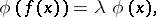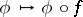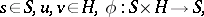# Schröder functional equation

(diff) ← Older revision | Latest revision (diff) | Newer revision → (diff)

The equation(a1)

whereis the unknown function andis a known real-valued function of a real variable. I.e. one asks for the eigenvalues and eigenfunctions of the composition operator (substitution operator). Sometimesis allowed to be a function itself.

One also considers the non-autonomous Schröder functional equationThe Schröder and Abel functional equations (see also Functional equation) have much to do with the translation functional equationwhereis a semi-group, which asks for something like a right action ofon, [a1], [a4].

The equation was formulated by E. Schröder, [a5], and there is an extensive body of literature.

How to Cite This Entry:
Schröder functional equation. Encyclopedia of Mathematics. URL: http://encyclopediaofmath.org/index.php?title=Schr%C3%B6der_functional_equation&oldid=15050
This article was adapted from an original article by M. Hazewinkel (originator), which appeared in Encyclopedia of Mathematics - ISBN 1402006098. See original article